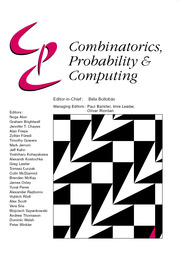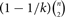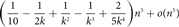Home
Hostname: page-component-59b7f5684b-vcb8f Total loading time: 0.387 Render date: 2022-09-30T16:33:55.897Z Has data issue: true Feature Flags: { "shouldUseShareProductTool": true, "shouldUseHypothesis": true, "isUnsiloEnabled": true, "useRatesEcommerce": false, "displayNetworkTab": true, "displayNetworkMapGraph": false, "useSa": true } hasContentIssue trueCombinatorics, Probability and Computing

# Minimizing the number of 5-cycles in graphs with given edge-density

Published online by Cambridge University Press:  09 October 2019

*
*Corresponding author. email: andrzej.dudek@wmich.edu

## Abstract

Motivated by the work of Razborov about the minimal density of triangles in graphs we study the minimal density of the 5-cycle C5. We show that every graph of order n and size$(1 - 1/k) \left( {\matrix{n \cr 2 }} \right)$, where k ≥ 3 is an integer, contains at least$$({1 \over {10}} - {1 \over {2k}} + {1 \over {{k^2}}} - {1 \over {{k^3}}} + {2 \over {5{k^4}}}){n^5} + o({n^5})$$
copies of C5. This bound is optimal, since a matching upper bound is given by the balanced complete k-partite graph. The proof is based on the flag algebras framework. We also provide a stability result. An SDP solver is not necessary to verify our proofs.

## MSC classification

Type
Paper
Information
Combinatorics, Probability and Computing , January 2020 , pp. 44 - 67
© Cambridge University Press 2019

## Access options

Get access to the full version of this content by using one of the access options below. (Log in options will check for institutional or personal access. Content may require purchase if you do not have access.)

## Footnotes

Supported in part by Simons Foundation grant 426894.

Supported in part by Simons Foundation grant 522400.

§

Supported in part by NSF grant DMS-1600390.

Supported in part by ERC grant 306493.

## References

Alon, N., Fischer, E., Krivelevich, M. and Szegedy, M. (2000) Efficient testing of large graphs. Combinatorica 20 451476.10.1007/s004930070001CrossRefGoogle Scholar
Balogh, J., Hu, P., Lidický, B. and Pfender, F. (2016) Maximum density of induced 5-cycle is achieved by an iterated blow-up of 5-cycle. European J. Combin . 52 (A) 4758.CrossRefGoogle Scholar
Borchers, B. (1999) CSDP, a C library for semidefinite programming. Optim. Methods Softw. 11 613623.CrossRefGoogle Scholar
Conlon, D. and Fox, J. (2013) Graph removal lemmas. In Surveys in Combinatorics 2013, Vol. 409 of London Mathematical Society Lecture Note Series, Cambridge University Press, pp. 149.Google Scholar
Erdös, P. (1984) On some problems in graph theory, combinatorial analysis and combinatorial number theory. In Graph Theory and Combinatorics (Cambridge, 1983), Academic Press, pp. 117.Google Scholar
Erdös, P. and Stone, A. H. (1946) On the structure of linear graphs. Bull. Amer. Math. Soc. 52 10871091.CrossRefGoogle Scholar
Frieze, A. and Karoński, M. (2016) Introduction to Random Graphs, Cambridge University Press.CrossRefGoogle Scholar
Grzesik, A. (2012) On the maximum number of five-cycles in a triangle-free graph. J. Combin. Theory Ser. B 102 10611066.CrossRefGoogle Scholar
Hatami, H., Hladký, J., Král’, D., Norine, S. and Razborov, A. (2013) On the number of pentagons in triangle-free graphs. J. Combin. Theory Ser. A 120 722732.CrossRefGoogle Scholar
Lidický, B. and Pfender, F. (2018) Pentagons in triangle-free graphs. European J. Combin . 74 8589.CrossRefGoogle Scholar
Liu, H., Pikhurko, O., Sharifzadeh, M. and Staden, K. (2019) Stability from symmetrisation arguments. Work in progress.Google Scholar
Liu, H., Pikhurko, O. and Staden, K. (2017) The exact minimum number of triangles in graphs of given order and size. arXiv:1712.00633Google Scholar
Lovász, L. (2012) Large Networks and Graph Limits, Colloquium Publications, American Mathematical Society.CrossRefGoogle Scholar
Lovász, L. and Simonovits, M. (1983) On the number of complete subgraphs of a graph, II. In Studies in Pure Mathematics (Erdös, P. et al., eds), Birkhäuser, pp. 459495.10.1007/978-3-0348-5438-2_41CrossRefGoogle Scholar
Lovász, L. and Szegedy, B. (2006) Limits of dense graph sequences. J. Combin. Theory Ser. B 96 933957.CrossRefGoogle Scholar
Lovász, L. and Szegedy, B. (2010) Testing properties of graphs and functions. Israel J. Math . 178 113156.CrossRefGoogle Scholar
Mantel, W. (1907) Problem 28. Winkundige Opgaven 10 6061.Google Scholar
Moon, J. W. and Moser, L. (1962) On a problem of Turán. Magyar Tud. Akad. Mat. Kutató Int. Közl. 7 283286.Google Scholar
Nikiforov, V. (2011) The number of cliques in graphs of given order and size. Trans. Amer. Math. Soc. 363 15991618.CrossRefGoogle Scholar
Nordhaus, E. A. and Stewart, B. M. (1963) Triangles in an ordinary graph. Canad. J. Math. 15 3341.CrossRefGoogle Scholar
Pikhurko, O. (2010) An analytic approach to stability. Discrete Math . 310 29512964.CrossRefGoogle Scholar
Pikhurko, O. and Razborov, A. (2017) Asymptotic structure of graphs with the minimum number of triangles. Combin. Probab. Comput. 26 138160.CrossRefGoogle Scholar
Pikhurko, O., Sliacan, J. and Tyros, K. (2019) Strong forms of stability from flag algebra calculations. J. Combin. Theory Ser. B 135 129178.CrossRefGoogle Scholar
Pippenger, N. and Golumbic, M. C. (1975) The inducibility of graphs. J. Combin. Theory Ser. B 19 189203.CrossRefGoogle Scholar
Razborov, A. (2007) Flag algebras. J. Symbolic Logic 72 12391282.CrossRefGoogle Scholar
Razborov, A. (2008) On the minimal density of triangles in graphs. Combin. Probab. Comput. 17 603618.CrossRefGoogle Scholar
Reiher, C. (2016) The clique density theorem. Ann. of Math. (2) 184 683707.CrossRefGoogle Scholar
Sidorenko, A. (1991) Inequalities for functionals generated by bipartite graphs. Diskret. Mat. 3 5065.Google Scholar
Turán, P. (1941) Eine Extremalaufgabe aus der Graphentheorie. Mat. Fiz. Lapok 48 436452.Google Scholar
1
Cited by

# Save article to Kindle

To save this article to your Kindle, first ensure coreplatform@cambridge.org is added to your Approved Personal Document E-mail List under your Personal Document Settings on the Manage Your Content and Devices page of your Amazon account. Then enter the ‘name’ part of your Kindle email address below. Find out more about saving to your Kindle.

Note you can select to save to either the @free.kindle.com or @kindle.com variations. ‘@free.kindle.com’ emails are free but can only be saved to your device when it is connected to wi-fi. ‘@kindle.com’ emails can be delivered even when you are not connected to wi-fi, but note that service fees apply.

Find out more about the Kindle Personal Document Service.

Minimizing the number of 5-cycles in graphs with given edge-density
Available formats
×

# Save article to Dropbox

To save this article to your Dropbox account, please select one or more formats and confirm that you agree to abide by our usage policies. If this is the first time you used this feature, you will be asked to authorise Cambridge Core to connect with your Dropbox account. Find out more about saving content to Dropbox.

Minimizing the number of 5-cycles in graphs with given edge-density
Available formats
×

# Save article to Google Drive

To save this article to your Google Drive account, please select one or more formats and confirm that you agree to abide by our usage policies. If this is the first time you used this feature, you will be asked to authorise Cambridge Core to connect with your Google Drive account. Find out more about saving content to Google Drive.

Minimizing the number of 5-cycles in graphs with given edge-density
Available formats
×
×